NCERT Solutions for Class 7 Maths Chapter 4 Simple Equations Ex 4.4 are part of NCERT Solutions for Class 7 Maths. Here we have given NCERT Solutions for Class 7 Maths Chapter 4 Simple Equations Ex 4.4.

 Board CBSE Textbook NCERT Class Class 7 Subject Maths Chapter Chapter 4 Chapter Name Simple Equations Exercise Ex 4.4 Number of Questions Solved 4 Category NCERT Solutions

## NCERT Solutions for Class 7 Maths Chapter 4 Simple Equations Ex 4.4

Question 1.
Set up equations and solve them to find the unknown numbers in the following cases:

(a) Add 4 to eight times a number; you get 60.
(b) One-fifth of a number minus 4 gives 3.
(c) If I take three-fourths of a number and add 3 to it, I get 21.
(d) I subtracted 11 from twice a number, the result was 15.
(e) Munna subtracts thrice the number of notebooks he has from 50, he finds the result to be 8.
(f) Ibenhal thinks of a number. If she adds 19 to it and divides the sum by 5, she will get 8.
(g) Anwar thinks of a number. If he takes away 7 from $$\frac { 5 }{ 2 }$$ of the number, the result is 23.

Solution: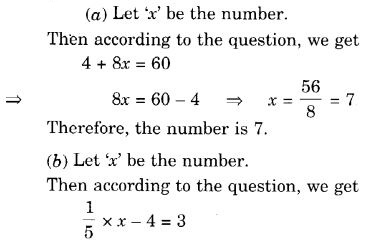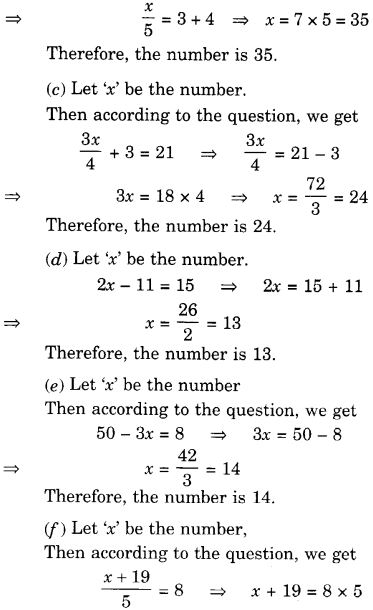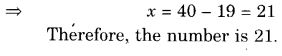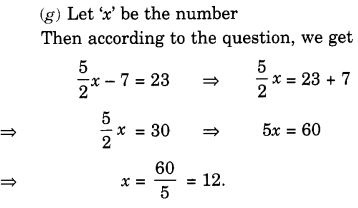Question 2.
Solve the following:
(a) The teacher tells the class that the highest marks obtained by a student in her class are twice the lowest mark plus 7. The highest score is 87. What is the lowest score?
(b) In an isosceles triangle, the base angles are equal. The vertex angle is 40°. What are the base angles of the triangle? (Remember, the sum of three angles of a triangle is 180°).
(c) Sachin scored twice as many runs as Rahul. Together, their runs fell two short of a double century. How many runs did each one score?
Solution: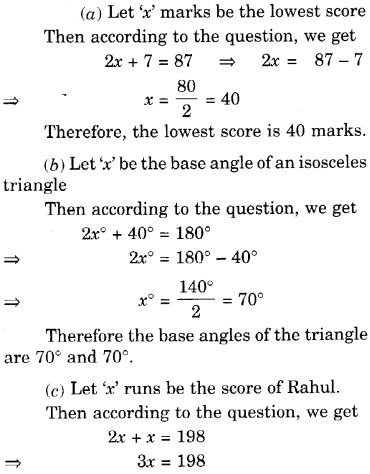Question 3.
Solve the following:

1. Irfan says that he has 7 marbles more than five times the marbles Parmit has. Irfan has 37 marbles. How many marbles does Parmit have?
2. Laxmi’s father is 49 years old. He is 4 years older than three times Laxmi’s age. What is Laxmi’s age?
3. The people of Sundargram planted trees in the village garden. Some of the trees were fruit trees. The number of non-fruit trees was two more than three times the number of fruit trees. What was the number of fruit trees planted if the number of non-fruit trees planted was 77?

Solution: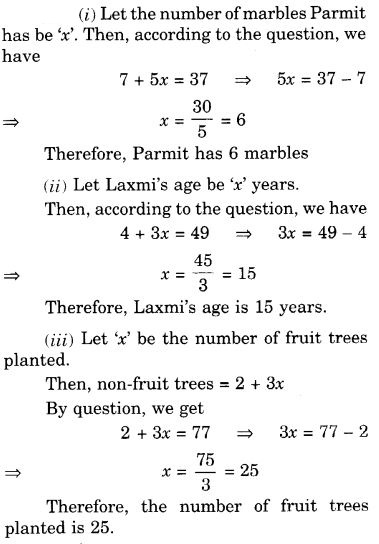Question 4.
Solve the following riddle :
I am a number, Tell me my identity!
Take me seven times over And add a fifty!
To reach a triple century You still need forty!
Solution:
Let ‘x’ be the number,
Then, according to the question, we get (x × 7) + 50 = 300 – 40
7x + 50 = 260
7x = 210
x = $$\frac { 210 }{ 7 }$$ = 30
So, the number is 30.

We hope the NCERT Solutions for Class 7 Maths Chapter 4 Simple Equations Ex 4.4 help you. If you have any query regarding NCERT Solutions for Class 7 Maths Chapter 4 Simple Equations Ex 4.4, drop a comment below and we will get back to you at the earliest.